GFG App
Open AppBrowser
Continue

# Class 8 NCERT Solutions – Chapter 4 Practical Geometry – Exercise 4.2

### Question 1: Construct the following quadrilaterals.

LI = 4 cm
IF = 3 cm
TL = 2.5 cm
LF = 4.5 cm
IT = 4 cm

Solution:

Steps of construction:

Step 1: Draw a line of 4 cm and label it as LI
Step 2: Taking I as the centre draw an arc of radius 3cm
Step 3: Taking L as the centre draw an arc of radius 4.5cm, so that both the arcs from I and L intersect each other and marks the intersection point as F. consider the fig below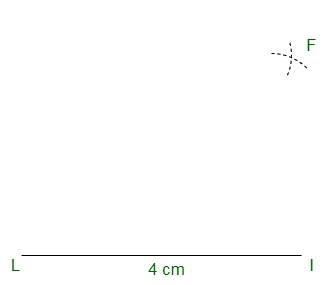Step 4: Join IF and LF
Step 5: Take L as centre draw an arc of radius 2.5 cm
Step 6: Take I as centre draw an arc of 4 cm to meet the previous arc and mark the intersection point as T.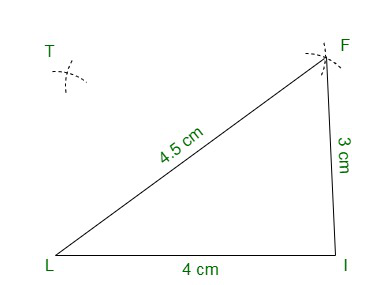Step 7: Join TF and TL
Step 8: Hence we have the required quadrilateral LIFT.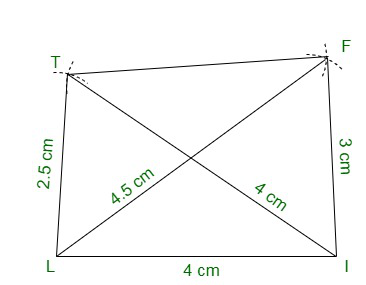OL = 7.5 cm
GL = 6 cm
GD = 6 cm
LD = 5 cm
OD = 10 cm

Solution:

Steps of construction:

Step 1: Draw a line OL of 7.5 cm
Step 2: Taking L as the centre draw an arc of 5 cm.
Step 3: Taking O as the centre draw an arc of 10 cm and let it intersect with the previous arc. Mark the intersection point as D as shown below: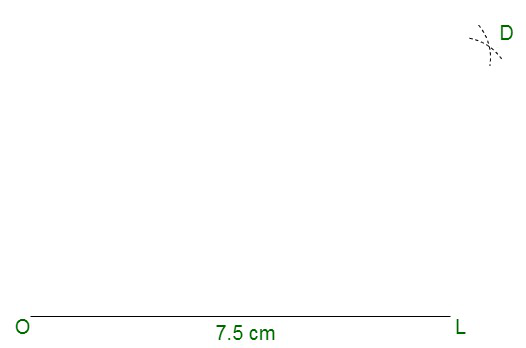Step 4: Join LD and OD.
Step 5: Take D as the centre and draw an arc of 6 cm.
Step 6: Now take L as the centre and drawn another arc of 6 cm that will intersect with the former arc at point G.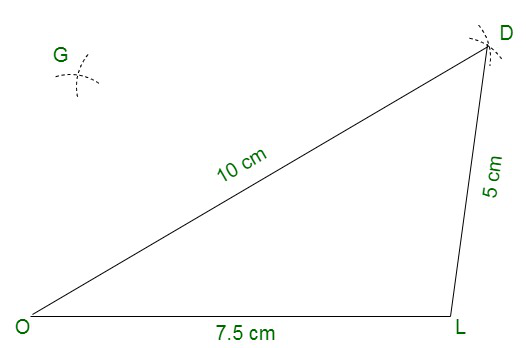Step 7: Join GL and GD.
Step 8: Hence we have the required quadrilateral GOLD.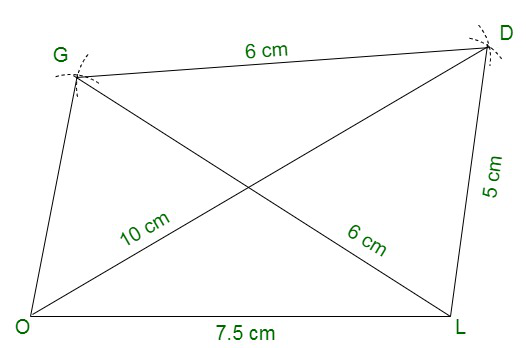(iii) Rhombus BEND
BN = 5.6 cm
DE = 6.5 cm

Solution:

Steps of construction:

Step 1: Draw a line BN of 5.6 cm
Step 2: Draw perpendicular bisector of BN and mark the bisector point as O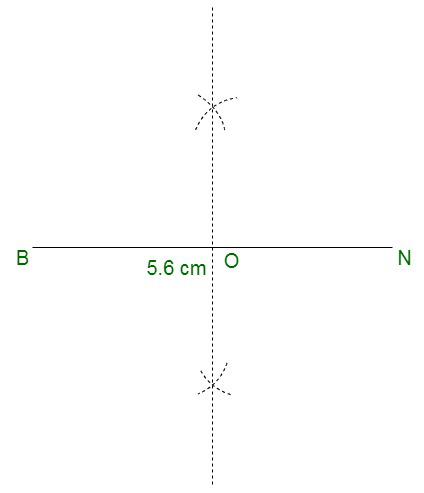Step 3:  Draw two arcs with centre O of radius  × DE =  × 6.5 cm = 3.25 cm and these arcs will meet the bisector at point D and E
2                2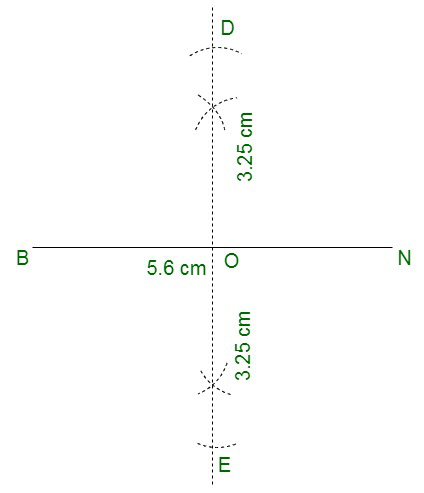Step 4: Join BE, EN, ND, and BD.
Step 5: Hence we have the required rhombus BEND.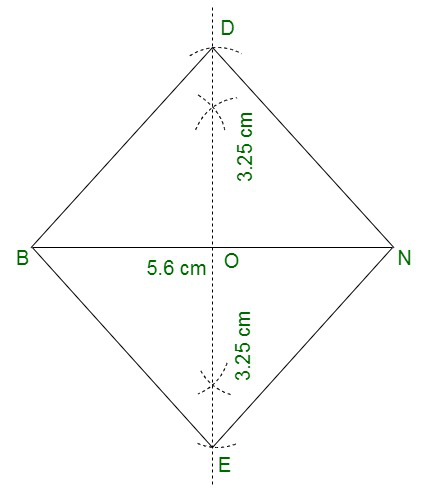My Personal Notes arrow_drop_up